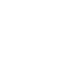# Question of the Day: June 6

If you are reading this in an email you received from me, do not click the link to sat.collegeboard.org below. Use the link to my website that is farther down on the email. If you are seeing this in my blog, dco the SAT Question of the Day by clicking on this link:

http://sat.collegeboard.org/practice/sat-question-of-the-day?src=R&questionId=20130606 (This link takes you to today’s question. If you use my archive, you will see the question related to my SAT explanation for that date.)

The answer is D.  This is a great question.  It can be done a lot of ways including 1) the way the SAT staff has explained it.  2) You could have done it algebraically by setting up the the milk price facts to begin with.  3) You could have said their answers using words instead of x, y, and z.  For example, using the right answer: “Starting with the price of two half-gallons (2x) and then subtracting the price of a gallon (y) and then subtracting the difference of the two prices (z) would be equal to zer0.”  All those work.  Here’s a couple of “fun” ways to do the problem.

Use my technique of changing the algebra to arithmetic.  Substitute numbers for the variables.  If a half-gallon cost 5 cents (x) and a gallon cost 8 cents (y), then the difference between two half-gallons and a gallon is 2 cents (z).  Substitute the real numbers you made up into the answers.  Only D works.  (BTW, use easy numbers like I just did.  You don’t have to use actual prices.  “The world of math is a world of patterns” strategy indicates you can use any numbers that follow the rules in the question.)

I did it that way but also saw another clever way to do the problem.  They told you that you had “2 half-gallons” which is 2x.  Because only Answers B and D have a 2x in them, they are the only possible right answers.  For example, Answer A has 1 half-gallon (x) and 2 gallons (2y) which makes no sense and can be eliminated.  We are down to B and D and the only difference between the two answers is whether to subtract or add z.  I had to subtract it since I was told that 2x was more than one gallon (y) and I would have to subtract the difference (z) to get to zero.  Shazaam!  That was fun.

Let’s see what we can do to demystify the ACT.

http://www.act.org/qotd/ (The ACT staff does not put a date on their questions so if you click on an archived blog, you’ll get today’s question and the old explanation. Sorry. The SAT staff has dated their questions; so, the archive is helpful. The ACT folks simply don’t do that.)

The answer is J.  Always remember the differences between their, there, and they’reThey’re is a contraction for “they are” and is easy to overlook during the rush of finishing the test on time.  Be careful when you see one of them underlined.  You need there in this sentence because it relates to a place.  You need a verb in the sentence; so, you have to pick Answer J.  Answer H drops the “she had as” and eliminates the verb which creates a different mistake: there’s no verb in the sentence.